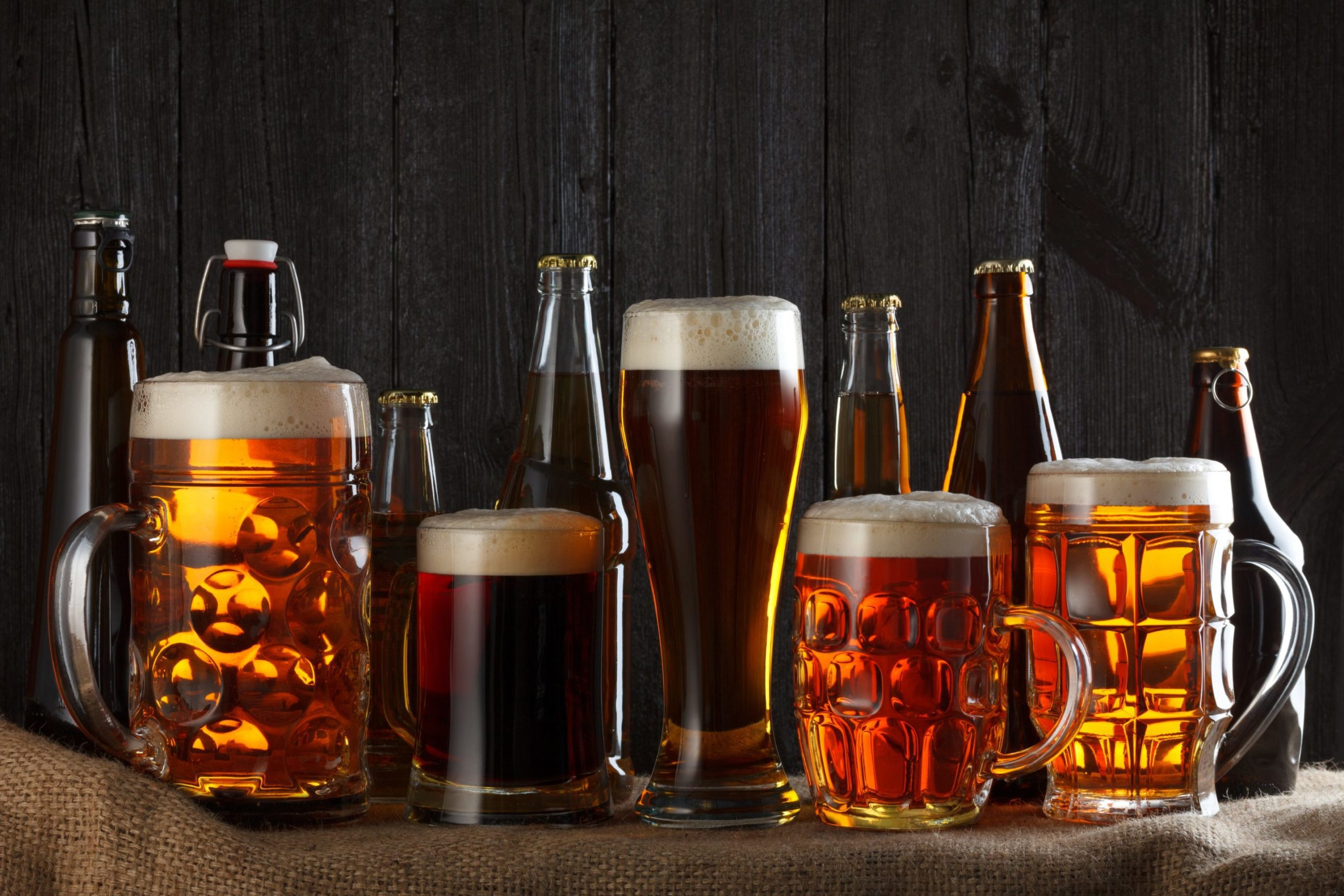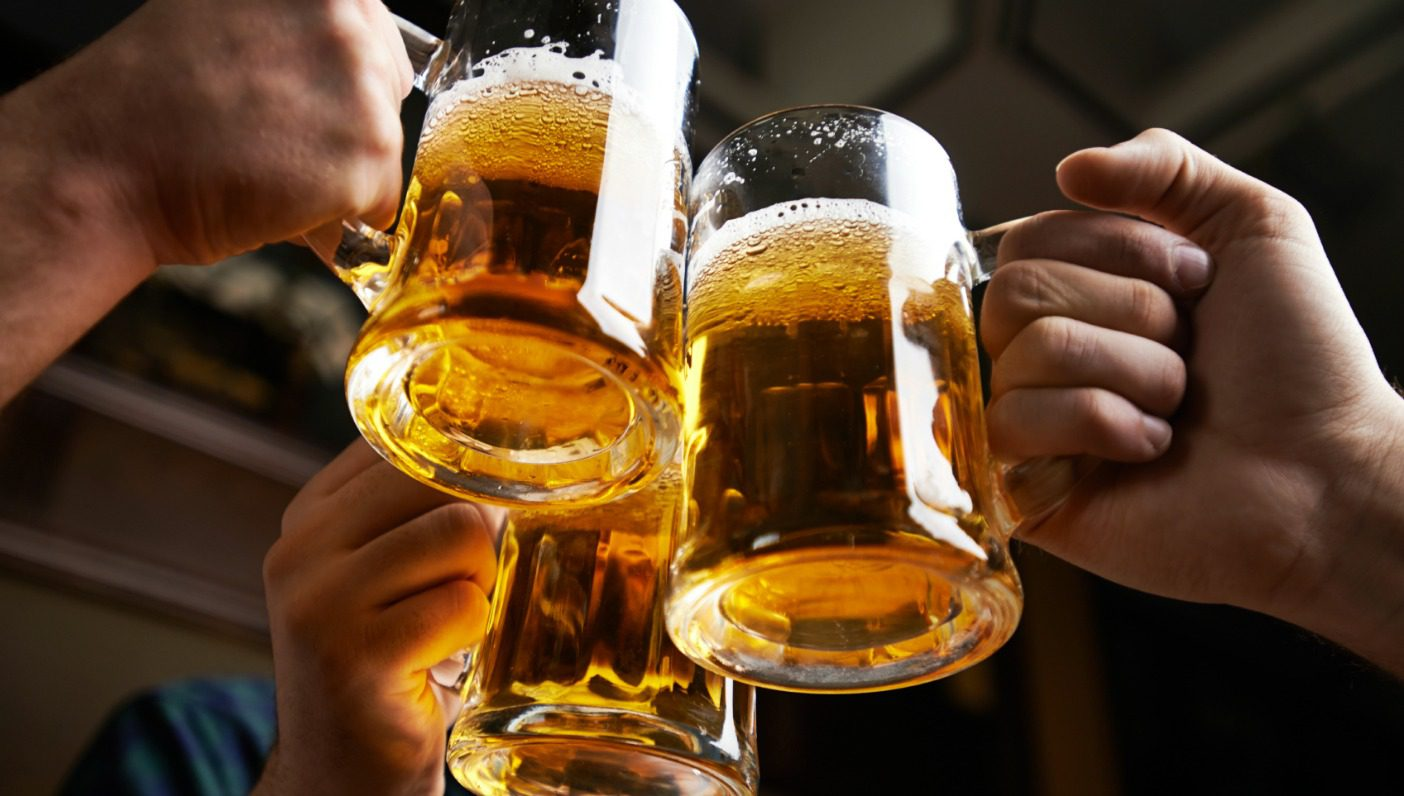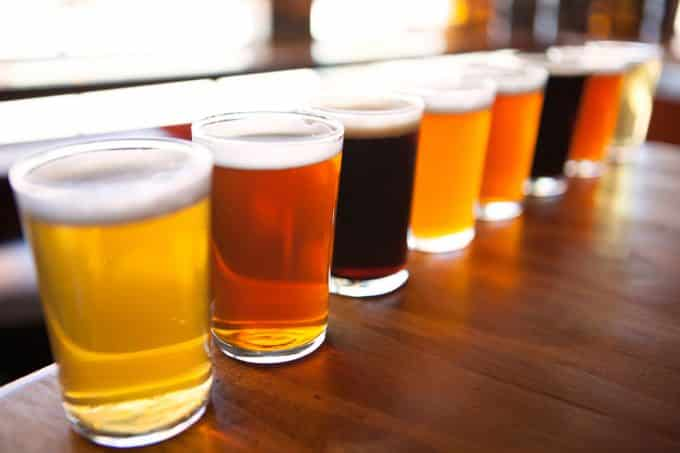# Calculating Alcohol %#### July 23, 2020

It is very simple to calculate the alcohol percentage of your brew by using your hydrometer.

All you need to do is take your Starting S.G. (specific gravity) & Final S.G. and calculate it according to the below formula:

Start S.G. – Final S.G. = (XXXX)

(XXXX) Divided by 7.36 = % Alc. Vol.

Add 0.5% to allow for alcohol produced from the priming sugar and you will have your overall % Alc. Vol.

Ie: 1038 – 1006 = 32

32 divided by 7.36 = 4.35 %

4.35% + .5% = 4.85% (Alc. Vol.)

If you keg your beer and gas it using Co2, don’t add the .5% as no further alcohol is produced.